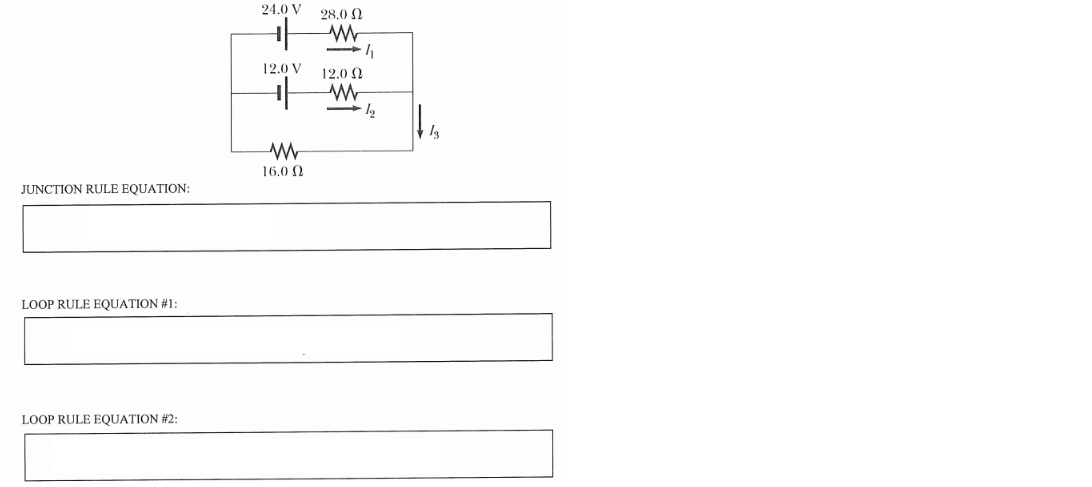# Problem: Present one junction and two loop rule equations that you could solve simultaneously to obtain values for the three currents, I1, I2 and I3, in the dc circuit shown below. Do not solve the equations!

###### FREE Expert Solution
89% (259 ratings)
###### Problem Details

Present one junction and two loop rule equations that you could solve simultaneously to obtain values for the three currents, I1, I2 and I3, in the dc circuit shown below. Do not solve the equations!